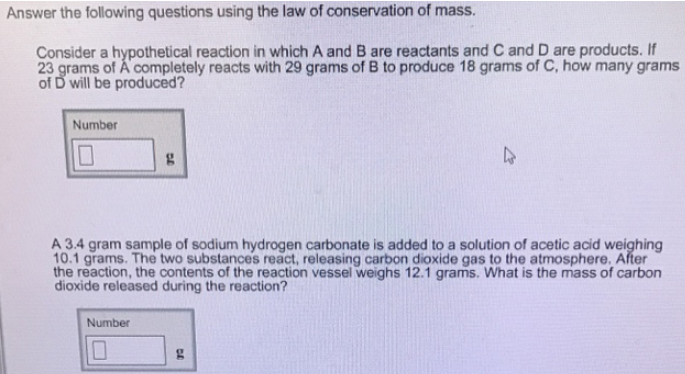# Problem: Answer the following questions using the law of conservation of mass.Consider a hypothetical reaction in which A and B are reactants and C and D are products. If 23 grams of A completely reacts with 29 grams of B to produce 18 grams of C, how many grams of D will be produced? A 3.4 gram sample of sodium hydrogen carbonate is added to a solution of acetic acid weighing 10.1 grams. The two substances react, releasing carbon dioxide gas to the atmosphere. After the reaction, the contents of the reaction vessel weighs 12.1 grams. What is the mass of carbon dioxide released during the reaction?

###### FREE Expert Solution
99% (115 ratings)###### Problem Details

Answer the following questions using the law of conservation of mass.

Consider a hypothetical reaction in which A and B are reactants and C and D are products. If 23 grams of A completely reacts with 29 grams of B to produce 18 grams of C, how many grams of D will be produced?

A 3.4 gram sample of sodium hydrogen carbonate is added to a solution of acetic acid weighing 10.1 grams. The two substances react, releasing carbon dioxide gas to the atmosphere. After the reaction, the contents of the reaction vessel weighs 12.1 grams. What is the mass of carbon dioxide released during the reaction?